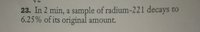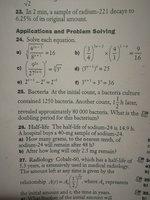# Math 30-1, exponential growth and decay

#### kris.yarmak123

##### Junior MemberHello. I am really terrible with the applications, and I would be very grateful if someone could help me to answer this question.
I have started with writing the equation y=0.9375^(x)
I checked if it passes through the point (0,1) and it does pass. Then I checked if it passes through the point (2, 0.0625) and it does not pass, so I tried to rewrite the equation as y=0.9375^(x/2) and y=0.9375^(2x)
....and none of the equations worked out

#### Jomo

##### Elite Member
OK, so you clearly are not getting the correct power. So stop guessing. Let the power be tx and then solve for t.

•topsquark

#### kris.yarmak123

##### Junior Member
OK, so you clearly are not getting the correct power. So stop guessing. Let the power be tx and then solve for t.
Y=0.9375^tx
0.0625=0.9375^2t
log(0.0625)/log(0.9375)=2t
42,96=2t
t=21.48
...like this?

#### Jomo

##### Elite Member
No need to ask me. Just check to see if the 2 points work. Let us know how you make out.

Also put parenthesis around tx !

#### kris.yarmak123

##### Junior Member
No need to ask me. Just check to see if the 2 points work. Let us know how you make out.

Also put parenthesis around tx !
Ok, thanks.
Y=0.9375^(tx)
0.0625=0.9375^(2t)
log(0.0625)/log(0.9375)=2t
42,96=2t
t=21.48

#### Jomo

##### Elite Member
Ok, thanks.
Y=0.9375^(tx)
0.0625=0.9375^(2t)
log(0.0625)/log(0.9375)=2t
42,96=2t
t=21.48
So does it work?

#### kris.yarmak123

##### Junior Member
So does it work?
No, I should get 30 as the answer, but I keep getting 21.48, so now I'm thinking what's wrong

#### OmarMohamedKhallaf

##### New member
I don't understand the question, but I think there is another part you didn't picture that asks for something and I think that this something is the decay rate.
If I'm right then you should start from here:
$$\displaystyle 6.25\%=100\%\times (1-x)^{2}\iff 0.0625=1\times(1-x)^{2}$$

#### kris.yarmak123

##### Junior Member
So does it work?
Ok I think I found a good way I guess
y=(1/2)^(2x)
It does pass through the points (0,1) and (2,0.0625)
I don't understand the question, but I think there is another part you didn't picture that asks for something and I think that this something is the decay rate.
If I'm right then you should start from here:
$$\displaystyle 6.25\%=100\%\times (1-x)^{2}\iff 0.0625=1\times(1-x)^{2}$$
There's no other part... Here's the whole pic of the questions#### Dr.Peterson

##### Elite Member
Ok I think I found a good way I guess
y=(1/2)^(2x)
It does pass through the points (0,1) and (2,0.0625)
There is no question in #23; it tells you something but doesn't say what you are to find!

Your new work suggests that maybe you are asked (in the instructions for that set of exercises?) for the half-life. (But that would be 30 seconds, and you didn't mention a unit in the answer!)

Please tell us what it is that you are trying to find; if there are instructions for the exercises, show us that, and if the answer is not just the number 30, tell us that, too.

#### kris.yarmak123

##### Junior Member
There is no question in #23; it tells you something but doesn't say what you are to find!

Your new work suggests that maybe you are asked (in the instructions for that set of exercises?) for the half-life. (But that would be 30 seconds, and you didn't mention a unit in the answer!)

Please tell us what it is that you are trying to find; if there are instructions for the exercises, show us that, and if the answer is not just the number 30, tell us that, too.

#### kris.yarmak123

##### Junior Member
How did you solve it.
I wrote 0.0625=(1/2)^(2/t) and got 0.50 min as the answer, so it's 30 sec

#### Dr.Peterson

##### Elite Member
Yes, that's right. There are several ways to determine this, depending on how you textbook presents it; but you've not only refused to tell us that you were asked for the half-life, you have never told us what form you are using for the equation, so it's really hard to help you.

One form I've seen is A = A0(1/2)^(t/T), where T is the half-life. Your exponent (as you correctly had it before) was 2t, which can be thought of as t/0.5, so the half-life is 0.5 minute = 30 seconds.

(Now that I look again, I see this form in your problem 27, with 5.7 as the half-life. So apparently I have correctly figured out what you are supposed to do.)

•kris.yarmak123

#### kris.yarmak123

##### Junior Member

Yes, that's right. There are several ways to determine this, depending on how you textbook presents it; but you've not only refused to tell us that you were asked for the half-life, you have never told us what form you are using for the equation, so it's really hard to help you.

One form I've seen is A = A0(1/2)^(t/T), where T is the half-life. Your exponent (as you correctly had it before) was 2t, which can be thought of as t/0.5, so the half-life is 0.5 minute = 30 seconds.

(Now that I look again, I see this form in your problem 27, with 5.7 as the half-life. So apparently I have correctly figured out what you are supposed to do.)
Yeah, I'm sorry about that, I was a little confused myself (